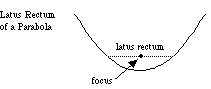index: click on a letter A B C D E F G H I J K L M N O P Q R S T U V W X Y Z A to Z index index: subject areas numbers & symbols sets, logic, proofs geometry algebra trigonometry advanced algebra & pre-calculus calculus advanced topics probability & statistics real world applications multimedia entrieswww.mathwords.com about mathwords website feedback

 Latus Rectum The line segment through a focus of a conic section, perpendicular to the major axis, which has both endpoints on the curve. Note: The length of a parabola's latus rectum is 4p, where p is the distance from the focus to the vertex.